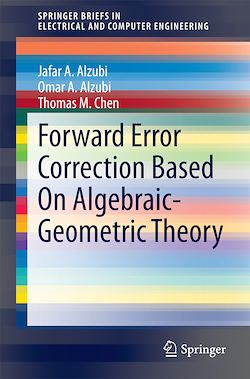# Forward Error Correction Based On Algebraic-Geometric Theory

-English
70 Pages

Description

This book covers the design, construction, and implementation of algebraic-geometric codes from Hermitian curves. Matlab simulations of algebraic-geometric codes and Reed-Solomon codes compare their bit error rate using different modulation schemes over additive white Gaussian noise channel model. Simulation results of Algebraic-geometric codes bit error rate performance using quadrature amplitude modulation (16QAM and 64QAM) are presented for the first time and shown to outperform Reed-Solomon codes at various code rates and channel models. The book proposes algebraic-geometric block turbo codes. It also presents simulation results that show an improved bit error rate performance at the cost of high system complexity due to using algebraic-geometric codes and Chase-Pyndiah’s algorithm simultaneously. The book proposes algebraic-geometric irregular block turbo codes (AG-IBTC) to reduce system complexity. Simulation results for AG-IBTCs are presented for the first time.

Subjects

##### Classwork

Informations